Home
Hostname: page-component-8bbf57454-gwkvl Total loading time: 0.495 Render date: 2022-01-23T04:23:46.321Z Has data issue: true Feature Flags: { "shouldUseShareProductTool": true, "shouldUseHypothesis": true, "isUnsiloEnabled": true, "metricsAbstractViews": false, "figures": true, "newCiteModal": false, "newCitedByModal": true, "newEcommerce": true, "newUsageEvents": true }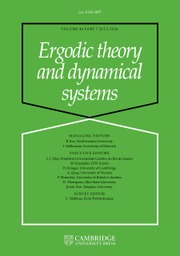Ergodic Theory and Dynamical Systems

Strongly ergodic equivalence relations: spectral gap and type III invariants

Published online by Cambridge University Press:  27 November 2017

Abstract

We obtain a spectral gap characterization of strongly ergodic equivalence relations on standard measure spaces. We use our spectral gap criterion to prove that a large class of skew-product equivalence relations arising from measurable$1$-cocycles with values in locally compact abelian groups are strongly ergodic. By analogy with the work of Connes on full factors, we introduce the Sd and$\unicode[STIX]{x1D70F}$ invariants for type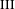$\text{III}$ strongly ergodic equivalence relations. As a corollary to our main results, we show that for any type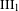$\text{III}_{1}$ ergodic equivalence relation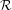${\mathcal{R}}$, the Maharam extension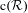$\text{c}({\mathcal{R}})$ is strongly ergodic if and only if${\mathcal{R}}$ is strongly ergodic and the invariant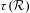$\unicode[STIX]{x1D70F}({\mathcal{R}})$ is the usual topology on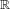$\mathbb{R}$. We also obtain a structure theorem for almost periodic strongly ergodic equivalence relations analogous to Connes’ structure theorem for almost periodic full factors. Finally, we prove that for arbitrary strongly ergodic free actions of bi-exact groups (e.g. hyperbolic groups), the Sd and$\unicode[STIX]{x1D70F}$ invariants of the orbit equivalence relation and of the associated group measure space von Neumann factor coincide.

Type
Original Article
Information
Ergodic Theory and Dynamical Systems , July 2019 , pp. 1904 - 1935

Access options

Get access to the full version of this content by using one of the access options below. (Log in options will check for institutional or personal access. Content may require purchase if you do not have access.)

References

Austin, T. and Moore, C. C.. Continuity properties of measurable group cohomology. Math. Ann. 356 (2013), 885937.CrossRefGoogle Scholar
Akemann, C. A. and Ostrand, P. A.. On a tensor product C -algebra associated with the free group on two generators. J. Math. Soc. Japan 27 (1975), 589599.CrossRefGoogle Scholar
Boutonnet, R., Houdayer, C. and Raum, S.. Amalgamated free product type III factors with at most one Cartan subalgebra. Compos. Math. 150 (2014), 143174.CrossRefGoogle Scholar
Boutonnet, R., Ioana, A. and Salehi Golsefidy, A.. Local spectral gap in simple Lie groups and applications. Invent. Math. 208 (2017), 715802.CrossRefGoogle Scholar
Brown, N. P. and Ozawa, N.. C -Algebras and Finite-Dimensional Approximations (Graduate Studies in Mathematics, 88) . American Mathematical Society, Providence, RI, 2008.CrossRefGoogle Scholar
Connes, A., Feldman, J. and Weiss, B.. An amenable equivalence relation is generated by a single transformation. Ergod. Th. & Dynam. Sys. 1 (1981), 431450.CrossRefGoogle Scholar
Connes, A. and Jones, V. F. R.. A II1 factor with two non-conjugate Cartan subalgebras. Bull. Amer. Math. Soc. (N.S.) 6 (1982), 211212.CrossRefGoogle Scholar
Connes, A.. Une classification des facteurs de type III. Ann. Sci. Éc. Norm. Supér. (4) 6 (1973), 133252.CrossRefGoogle Scholar
Connes, A.. Almost periodic states and factors of type III1 . J. Funct. Anal. 16 (1974), 415445.CrossRefGoogle Scholar
Connes, A.. Outer conjugacy classes of automorphisms of factors. Ann. Sci. Éc. Norm. Supér. (4) 8 (1975), 383419.CrossRefGoogle Scholar
Connes, A.. Classification of injective factors. Cases II1 , II , III𝜆 , 𝜆≠1. Ann. of Math. (2) 74 (1976), 73115.CrossRefGoogle Scholar
Feldman, J. and Moore, C. C.. Ergodic equivalence relations, cohomology, and von Neumann algebras. I, II. Trans. Amer. Math. Soc. 234 (1977), 289324; 325–359.CrossRefGoogle Scholar
Haagerup, U.. Connes’ bicentralizer problem and uniqueness of the injective factor of type III1 . Acta Math. 69 (1986), 95148.Google Scholar
Houdayer, C. and Isono, Y.. Bi-exact groups, strongly ergodic actions and group measure space type III factors with no central sequence. Comm. Math. Phys. 348 (2016), 9911015.CrossRefGoogle Scholar
Houdayer, C., Marrakchi, A. and Verraedt, P.. Fullness and Connes’$\unicode[STIX]{x1D70F}$ invariant of type$\text{III}$ tensor product factors. Preprint, 2016, arXiv:1611.07914.Google Scholar
Jones, V. F. R.. Central sequences in crossed products of full factors. Duke Math. J. 49 (1982), 2933.CrossRefGoogle Scholar
Krieger, W.. On non-singular transformations of a measure space. I, II. Z. Wahrscheinlichkeitstheorie und Verw. Gebiete 11 (1969), 8397; 98–119.CrossRefGoogle Scholar
Krieger, W.. On ergodic flows and the isomorphism of factors. Math. Ann. 223 (1976), 1970.CrossRefGoogle Scholar
Kechris, A. S. and Tsankov, T.. Amenable actions and almost invariant sets. Proc. Amer. Math. Soc. 136 (2008), 687697.CrossRefGoogle Scholar
Marrakchi, A.. Spectral gap characterization of full type III factors. J. Reine Angew. Math. to appear. Published online 12 January 2017, doi:10.1515/crelle-2016-0071.Google Scholar
Moore, C. C.. Group extensions and cohomology for locally compact groups. III. Trans. Amer. Math. Soc. 221 (1976), 133.CrossRefGoogle Scholar
Ozawa, N.. Solid von Neumann algebras. Acta Math. 192 (2004), 111117.CrossRefGoogle Scholar
Ozawa, N.. A Kurosh type theorem for type II1 factors. Int. Math. Res. Not. IMRN (2006), Art. ID 97560.CrossRefGoogle Scholar
Ozawa, N.. An example of a solid von Neumann algebra. Hokkaido Math. J. 38 (2009), 557561.CrossRefGoogle Scholar
Ozawa, N.. A remark on fullness of some group measure space von Neumann algebras. Compos. Math. 152 (2016), 24932502.CrossRefGoogle Scholar
Popa, S.. Some computations of 1-cohomology groups and construction of non orbit equivalent actions. J. Inst. Math. Jussieu 5 (2006), 309332.CrossRefGoogle Scholar
Schmidt, K.. Asymptotically invariant sequences and an action of SL(2, ℤ) on the 2-sphere. Israel J. Math. 37 (1980), 193208.CrossRefGoogle Scholar
Schmidt, K.. Amenability, Kazhdan’s property T, strong ergodicity and invariant means for ergodic group-actions. Ergod. Th. & Dynam. Sys. 1 (1981), 223236.CrossRefGoogle Scholar
Skandalis, G.. Une notion de nucléarité en K-théorie (d’après J. Cuntz). J. K-Theory 1 (1988), 549573.CrossRefGoogle Scholar
Takesaki, M.. Theory of operator algebras. II. Encyclopaedia of Mathematical Sciences. Vol. 125 (Operator Algebras and Non-commutative Geometry, 6) . Springer, Berlin, 2003.Google Scholar
Takesaki, M.. Theory of operator algebras. III. Encyclopaedia of Mathematical Sciences. Vol. 127 (Operator Algebras and Non-commutative Geometry, 8) . Springer, Berlin, 2003.Google Scholar
Vaes, S. and Verraedt, P.. Classification of type III Bernoulli crossed products. Adv. Math. 281 (2015), 296332.CrossRefGoogle Scholar
Vaes, S. and Wahl, J.. Bernoulli actions of type$\text{III}_{1}$ and$\text{L}^{2}$ -cohomology. Preprint, 2017, arXiv:1705.00439.CrossRefGoogle Scholar
Zimmer, R.. Extensions of ergodic group actions. Illinois J. Math. 20 (1976), 373409.CrossRefGoogle Scholar
Zimmer, R.. Ergodic theory and semisimple groups. Monographs in Mathematics. Vol. 81. Birkhäuser, Basel, 1984.Google Scholar

1
Cited by

Send article to Kindle

Note you can select to send to either the @free.kindle.com or @kindle.com variations. ‘@free.kindle.com’ emails are free but can only be sent to your device when it is connected to wi-fi. ‘@kindle.com’ emails can be delivered even when you are not connected to wi-fi, but note that service fees apply.

Find out more about the Kindle Personal Document Service.

Strongly ergodic equivalence relations: spectral gap and type III invariants
Available formats
×

Send article to Dropbox

To send this article to your Dropbox account, please select one or more formats and confirm that you agree to abide by our usage policies. If this is the first time you use this feature, you will be asked to authorise Cambridge Core to connect with your <service> account. Find out more about sending content to Dropbox.

Strongly ergodic equivalence relations: spectral gap and type III invariants
Available formats
×

To send this article to your Google Drive account, please select one or more formats and confirm that you agree to abide by our usage policies. If this is the first time you use this feature, you will be asked to authorise Cambridge Core to connect with your <service> account. Find out more about sending content to Google Drive.

Strongly ergodic equivalence relations: spectral gap and type III invariants
Available formats
×
×